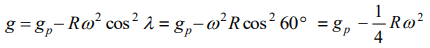## Gravitation Questions and Answers Part-6

1.The moon's radius is 1/4 that of the earth and its mass is 1/80 times that of the earth. If g represents the acceleration due to gravity on the surface of the earth, that on the surface of the moon is
a) g/4
b) g/5
c) g/6
d) g/8

Explanation:2. R is the radius of the earth and $\omega$ is its angular velocity and $g_{p}$ is the value of g at the poles. The effective value of g at the latitude $\lambda=60^{0}$ will be equal to
a) $g_{p}-\frac{1}{4}R\omega^{2}$
b) $g_{p}-\frac{3}{4}R\omega^{2}$
c) $g_{p}-R\omega^{2}$
d) $g_{p}+\frac{1}{4}R\omega^{2}$

Explanation:3. The depth d at which the value of acceleration due to gravity becomes $\frac{1}{n}$ times the value at the surface, is [R = radius of the earth]
a) $\frac{R}{n}$
b) $R\left(\frac{n-1}{n}\right)$
c) $\frac{R}{n^{2}}$
d) $R\left(\frac{n}{n+1}\right)$

Explanation:4. At what height over the earth's pole, the free fall acceleration decreases by one percent (assume the radius of earth to be 6400 km)
a) 32 km
b) 80 km
c) 1.253 km
d) 64 km

Explanation:5. The diameters of two planets are in the ratio 4 : 1 and their mean densities in the ratio 1 : 2. The acceleration due to gravity on the planets will be in ratio
a) 1 : 2
b) 2 : 3
c) 2 : 1
d) 4 : 1

Explanation:6. At what altitude in metre will the acceleration due to gravity be 25% of that at the earth's surface (Radius of earth = R metre)
a) $\frac{1}{4}R$
b) R
c) $\frac{3}{8}R$
d) $\frac{R}{2}$

Explanation:7. If the angular speed of the earth is doubled, the value of acceleration due to gravity (g) at the north pole
a) Doubles
b) Becomes half
c) Remains same
d) Becomes zero

Explanation: Acceleration due to gravity at poles is independent of the angular speed of earth.

8. At the surface of a certain planet, acceleration due to gravity is one-quarter of that on earth. If a brass ball is transported to this planet, then which one of the following statements is not correct
a) The mass of the brass ball on this planet is a quarter of its mass as measured on earth
b) The weight of the brass ball on this planet is a quarter of the weight as measured on earth
c) The brass ball has the same mass on the other planet as on earth
d) The brass ball has the same volume on the other planet as on earth

Explanation: Mass of the ball always remain constant. It does not depend upon the acceleration due to gravity

9.Weight of 1 kg becomes 1/6 on moon. If radius of moon is $1.768\times10^{6 }m$   , then the mass of moon will be
a) $1.99\times10^{30}kg$
b) $7.56\times10^{22}kg$
c) $5.98\times10^{24}kg$
d) $7.65\times10^{22}kg$